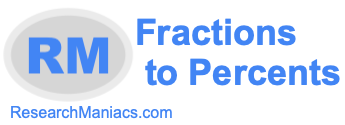What is 57/80 as a percent?Converting a fraction such as 57/80 into a percent is pretty easy.

All you have to do is divide the numerator by the denominator and then multiply that result with 100 like so:

(Numerator/Denominator)*100

When you enter 57/80 into the above formula, you get (57/80)*100 which calculates to:

71.25%

Note: When Research Maniacs calculated 57/80 as a percent, we rounded the answers to nine digits after the decimal point if necessary.

Fractions to Percents
Now you know 57/80 as a percent. To convert another fraction to percent, enter the fraction below and press "Make Percent"

/

What is 57/81 as a percent?
You may also be interested in the next fraction we converted to percent.